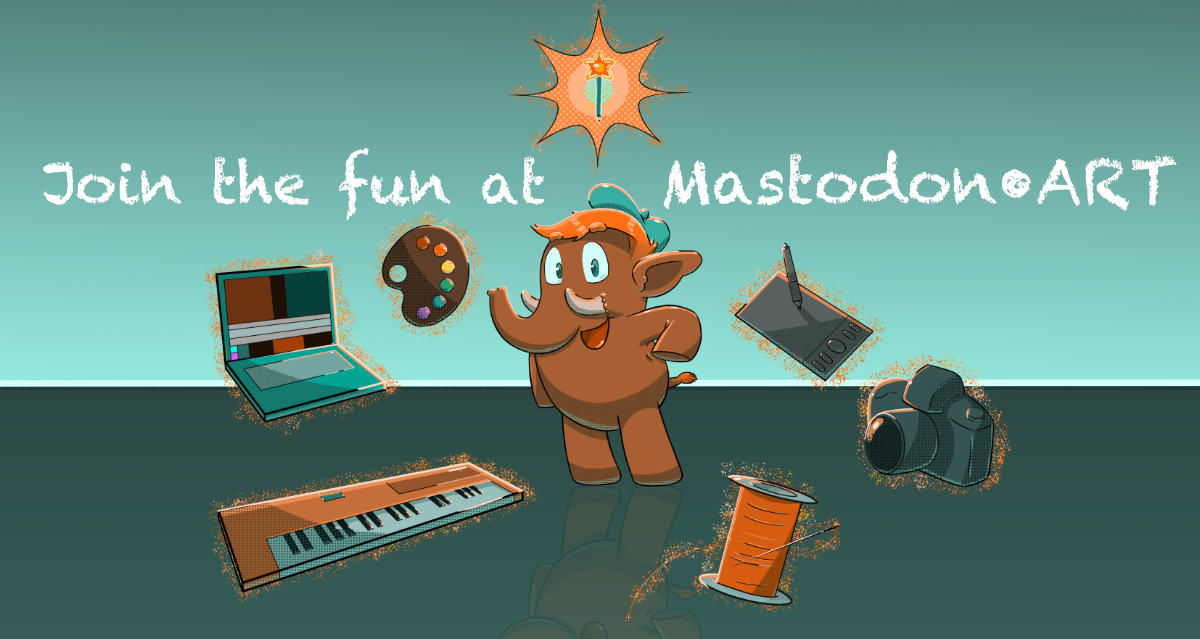v=130pal({-4,12,14,-9,-7,v},1)f=sin::_::cls()e=t()/9for i=e*90%9,256,9do
line(0,i,v,i-v,6)end
for j=1,9do
for s=0,6do
d=j*9o=f(e+j/20)/3a=o+s/6x=cos(a)*d+64y=f(a)*d+64for k=0,17do
r=1+(5+f(e)*4+k/4)%6p=r%1g=1-p
if(r-p==s)circ(x*p+c*g,y*p+z*g,2,k/3)end
c=x
z=y
end
end
flip()goto _

Spiral Maze
9" x 12"
Red and blue Micron on Bristol

Experimenting more with a maze pattern I've used a few times.

This drawing and others available at shop.andymakes.com/

t=0
draw=_=>{t+=.01
createCanvas(w=400,w)
a=cos(t)
b=sin(t)
strokeWeight(2)
for(x=0;x<w;x+=9){a=PI/4+sin(t)/4
for(y=h=noise(x/w,a,b)*w*.6;y>0;y-=5)d()
a+=PI/2
for(y=i=w/2+noise(x/w+a,b,9)*w*.4;y<w;y+=5)d()
a=0
for(y=h;y<i;y+=5)d()}}
d=_=>{line(x,y,x+cos(a)*13,y+sin(a)*13)}//#p5t

Pennies
9" x 12"
Micron on Bristol

Really enjoying this shadow algorithm. I Like it best when there's only a little of it.

This drawing and many others available at shop.andymakes.com/

I thought this tweet-length processing sketch posted by @Hau_kun would make a great drawing and I was right!

twitter.com/Hau_kun/status/139

I re-wrote it in C++ but beyond the noise function behaving a little differently, the output is identical to their source code.

I made this for my plotter, but you can use it with any device that accepts G-code. Laser cutters and CNC machines will probably require a little tweaking, but the basics should work.

My AxiDraw plotter doesn't read G-code out of the box, but I have a tool for that!

github.com/andymasteroffish/ax

After some cleanup & creating 8 example projects, I'm ready to release ofxGCode!

I've built this openFrameworks library up over the last year for all of my plotter work! A quick way to generate G-code in C++

github.com/andymasteroffish/of

--bad arteries
pal({8,14,2,0},1)
::_::
cls()
e=t()
s=20
for i=1,75 do
srand(i)
p=(e+i/(75+sin(e/2)*35))%1
c=1+rnd(3)
for k=rnd(s),180,s do
d=k+s*p*p*p
a=i/75+d/500
x=64+cos(a)*d
y=64+sin(a)*d
circ(x,y,d/10,c)
end
end
flip()
goto _
--

cls()
::🖌::
x=rnd(128)y=rnd(128)
if(pget(x,y)==0and x%2<1)pset(x,y-1,0)
if(pget(x,y)==0and y%2<1)pset(x+1,y,0)
if(pget(x,y)==7and x%2>1)pset(x,y+1,7)
for i=0,1 do
d=73-i*18a=-t()/15+i*.5s=12-4*i
circfill(64+cos(a)*d,65+sin(a)*d,s,7*i)
end
goto 🖌
--

Text Cascade
12"x9"
Rollerball on Bristol

Messing around with Hershey fonts. Every mark on this drawing is one of the following ASCII symbols: <*%/X->

You can buy this and other drawings at shop.andymakes.com/

t=0
draw=_=>{createCanvas(w=400,w)
colorMode(HSB,n=99)
p=stroke
l=line
s=PI/3
t+=.015
for(i=0;i<=w;i+=200)
for(y=0;y<w;y++)
for(k=0;k<TAU;k+=s){x=i+cos(a=k+t+noise(y/200,t/9,i/40)+sin(y/n+t))*n
c=i+cos(a+s)*n
if(x>c){p((i/8+y/9+t*9)%n,n,x-c)
l(x,y,c,y)
p(0)
l(c,y,c+2,y)}}}
//#p5t

Hard Candy Variations
34"x22"
Micron on Bristol

I was commissioned to make this giant plot of my Hard Candies drawing! It's a quadriptic made up of 17"x11" sheets.

Top left is "normal" with the other sheets adding variation as they move along the X and Y axis.

--shimmer stripes
pal({12,137,13,10,9},1)
::_::
i=1
for c=-1,1,2 do
x=rnd(128)
y=rnd(128)
pset(x,y,i+((y+x/2+t()*5*c-t()*10)/20)%2)
x=rnd(128)
y=rnd(128)
n=((y/2+x+t()*5*c+t()*10)/5)%10
if(n<2)pset(x,y,2+i+n)
i+=1
end
goto _
--

--wobble prism
pal({136,11,3,137,3,11},1)
::_::
e=t()
for i=1,350 do
e-=.001
s=i/2
a=s/99+(e+i/200)/5
x=64+cos(a)*5
y=64+sin(a)*5
line()
for k=0,3 do
a=e/20+k/3
line(x+cos(a)*s,y+sin(a)*s,1+(i*.05-t()*2)%6)
end
end
flip()
goto _
--

Lost Planet

Expanded on my plasma/noise sketch from last night

--lost planet
w=128s=sin
pal({131,12,131,9,10,14,136,137},1)
::_::
x=rnd(w)
y=rnd(w)
e=t()
a=0
c=x
r=y
if sqrt((x-64)^2+(y-64)^2)<40 then
a=2
e/=3
c=w-x
r=w-y
end
p=3+(s(x/160+e/2)+cos(y/220+s(e/8))+s(x/w)/2+s(e*cos(y/72-e))/5+cos(x/70+y/40+e)/5)*2
if(p<1)p=7
pset(c,r,p+a)
goto _

--sun spots
pal({131,12,9,10,14,136,137},1)
::_::
for k=0,9999 do
x=rnd(128)
y=rnd(128)
p=2+(sin(x/160+t()/2)+cos(y/220+sin(t()/8))+sin(x/120)/2+sin(t()*cos(y/72-t()))/5+cos(x/70+y/40+t())/5)*2
if(p<1)p=7
pset(x,y,p)
end
goto _
--

Getting ready for the next round of the gallery at
Wonderville NYC!

We want to show your carts!

This rotating gallery of 280 char or less programs is curated by me & Death By Audio Arcade.

Super short submission form: docs.google.com/forms/d/e/1FAI

(code in reply, of course)

--star crossed
pal({7,135,10,9,137},1)l=line
cls()s=sin
::_::
for i=-1,1,2do
for k=1,4do
l()
c=s(t()/10)*63*i
r=s(t()/10)*63
l(63+c,63+r)
d=1
while d<160do
a=t()/10+k/4
n=1+(s(a*2)+cos(s(a/4))+s(a*3.1))/3
d+=9*n
l(63+c+cos(a)*d,63+r+s(a)*d,1+d/3%5)
end
end
end
goto _
--

pal({2,136,8,14,137},1)
cls()
r=rnd
::_::
for d=1,10do
f=(d*11+t()*11)%110
a=t()/5
circ(63+cos(a)*f,63+sin(a)*f,min(f,4),1+d%5)
end
a=t()/30
for i=0,999do
x=r(128)y=r(128)c=pget(x-x%x,y-y%y)d=r(6)
if(c>0)pset(x+sin(a)*d,y+cos(a)*d,c)
end
flip()goto _
--

Show olderMastodon.ART — Your friendly creative home on the Fediverse! Interact with friends and discover new ones, all on a platform that is community-owned and ad-free. Admin: @Curator. Moderators: @EmergencyBattle, @ScribbleAddict, @TapiocaPearl, @Otherbuttons, @katwylder